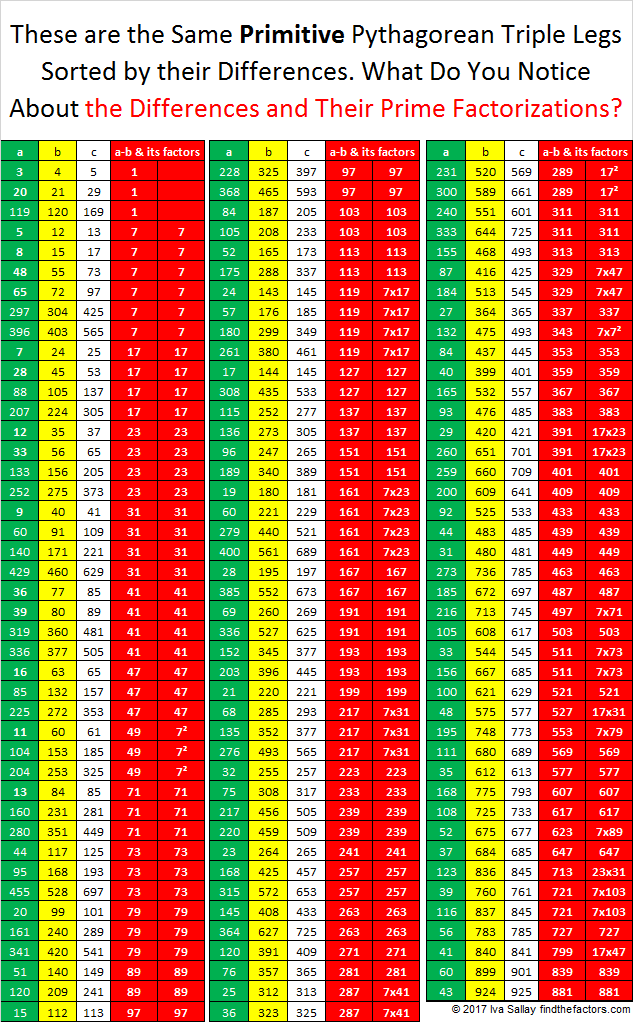# 863 These Primitive Pythagorean Triples Have Some Terrific Legs!

We’ve seen that the sum of the hypotenuse of a primitive Pythagorean triple and either of its legs is either a perfect square or two times a perfect square. What about just the legs themselves? Is there anything special about their sums? I think so. I think primitive Pythagorean triples have some terrific legs! Look at this chart and see if you agree.If a sum is a prime number, there is only one way to get that sum. If the sum is repeated, then its prime factorization only uses prime numbers already on the list.

I’m not as impressed with the differences of the legs, but it is interesting that the same prime numbers show up:863 is the sum of consecutive primes several ways:

• 863 is the sum of the fifteen prime numbers from 29 to 89.
• 863 is the sum of the thirteen prime numbers from 41 to 97.
• 107 + 109 + 113 + 127 + 131 + 137 + 139 = 863; that’s seven consecutive primes.
• 163 + 167+ 173 + 179 + 181 = 863; that’s five consecutive primes.

863(863 + 6) = 749,947, a palindrome. Thank you OEIS.org for that fun fact.

• 863 is a prime number.
• Prime factorization: 863 is prime.
• The exponent of prime number 863 is 1. Adding 1 to that exponent we get (1 + 1) = 2. Therefore 863 has exactly 2 factors.
• Factors of 863: 1, 863
• Factor pairs: 863 = 1 × 863
• 863 has no square factors that allow its square root to be simplified. √863 ≈ 29.37686How do we know that 863 is a prime number? If 863 were not a prime number, then it would be divisible by at least one prime number less than or equal to √863 ≈ 29.4. Since 863 cannot be divided evenly by 2, 3, 5, 7, 11, 13, 17, 19, 23, or 29, we know that 863 is a prime number.

This site uses Akismet to reduce spam. Learn how your comment data is processed.